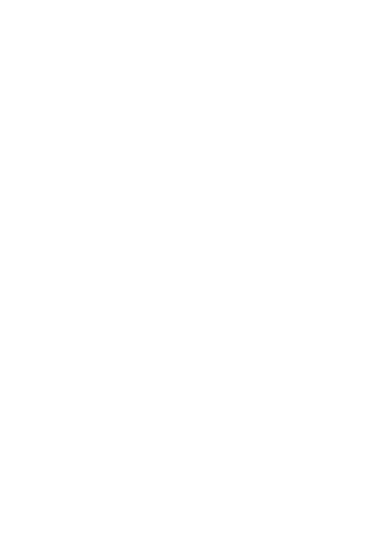JEE## Introduction

Differential calculus is concerned with the rate at which one quantity changes in relation to another. You can also think of it as a study of quantity change rates. For instance, velocity is the rate at which a distance changes with respect to time in a specific direction. If f(x) is a function, the differential equation is f'(x) = dy/dx, where f'(x) is the function's derivative, y is the dependent variable, and x is the independent variable.

### Important Terms in Differential Calculus

• Function

• Variable

• Domain and Range

• Limits

• Continuity

• Differentiability

• Differentiation formulae

• Tangent and Normal

• Approximation

• Rate measure

• Maxima and Minima

• Differential Equation

### Limit

In calculus, the limit is extremely significant. In calculus, limits are used to define continuity, integrals, and derivatives. A function's limit is defined as follows:

Let's say the function "f" is defined on an open interval containing some numbers, such as "a," with the exception of "a" itself. The limit of a function f(x) is written as: $\begin{array}{l}\lim_{x\rightarrow a}f(x)= L\end{array}$ ,iff  given e > 0, there exists d > 0 such that 0 < |x – a| < d implies that |f(x) – L| < e It means that the limit f(x) as “x” approaches “a” is “L”.

### Continuity

Continuity of a function states the characteristics of the function and its functional value. A function is said to be continuous if the curve has no missing points or breaking points in a given interval or domain, that is the curve is continuous at every point in its domain.

A function f(x) is known as a continuous function at a point x = a, in its domain if the following listed three conditions are satisfied-

1. f (a) exists which means that the value of f (a) is finite.

2. $\displaystyle \lim_{x \to a} f(x)$ exists, that is the right-hand limit = left-hand limit, and both R.H.S and L.H.S are finite.

3. $\displaystyle \lim_{x \to a} f(x)$  =  f (a)

A function f(x) is said to be continuous in the given interval I that is equal to [x1, x2] only if the three conditions listed above are satisfied for every point in the given interval I.

### Formal Definition of Continuity

A function is said to be continuous in the closed interval (a,b) if:

1. f is continuous in (a, b)

2. $\displaystyle \lim_{x \to a^{+}} f(x) = f(a)$

3. $\displaystyle \lim_{x \to a^{1}} f(x)= f(a)$

A function is said to be continuous in the open interval (a, b) if, f (x) is going to be continuous within the unbounded interval (a, b) if at any point within the given interval the function is continuous.

### Differentiability

Function f(x) is said to be differentiable at the point x = a and if the derivative of the function f ‘(a) exists at every point in its given domain.

Differentiability Formula

The differentiability formula is defined by -

f’(a) = $\frac{f(a+h)-f(a)}{h}$

If a function is continuous at a particular point then a function is said to be differentiable at any point x = a in its domain. The vice versa of this is not always true.

### Derivative

1.

$\frac{d}{dx}\left ( sin \, x \right )$

$cos \, x$

2.

$\frac{d}{dx}\left ( cos \, x \right )$

$- sin \, x$

3.

$\frac{d}{dx}\left ( tan \, x \right )$

$sec^{2}x$

4.

$\frac{d}{dx}\left ( cot \, x \right )$

$- cosec^{2}x$

5.

$\frac{d}{dx}\left ( sec \, x \right )$

$sec \, x.\, tan \, x$

6.

$\frac{d}{dx}\left ( cosec \, x \right )$

$- cosec \, x.\, cot \, x$

7.

$\frac{d}{dx}\left ( sin^{-1}x \right )$

$\frac{1}{\sqrt{1-x^{2}}}$

8.

$\frac{d}{dx}\left ( cos^{-1}x \right )$

$-\frac{1}{\sqrt{1-x^{2}}}$

9.

$\frac{d}{dx}\left ( tan^{-1}x \right )$

$\frac{1}{1+x^{2}}$

10.

$\frac{d}{dx}\left ( cot^{-1}x \right )$

$- \frac{1}{1+x^{2}}$

11.

$\frac{d}{dx}\left ( sec^{-1}x \right )$

$\frac{1}{\left|x \right|\sqrt{x^{2}-1}}$

12.

$\frac{d}{dx}\left ( cosec^{-1}x \right )$

$-\frac{1}{\left|x \right|\sqrt{x^{2}-1}}$

13.

$\frac{d}{dx}\left ( a^{x} \right )$

$a^{x}\, lnx$

14.

$\frac{d}{dx}\left ( e^{x} \right )$

$e^{x}$

15.

$\frac{d}{dx}\left ( ln\, x \right )$

$\frac{1}{x}$

### Tangent and Normal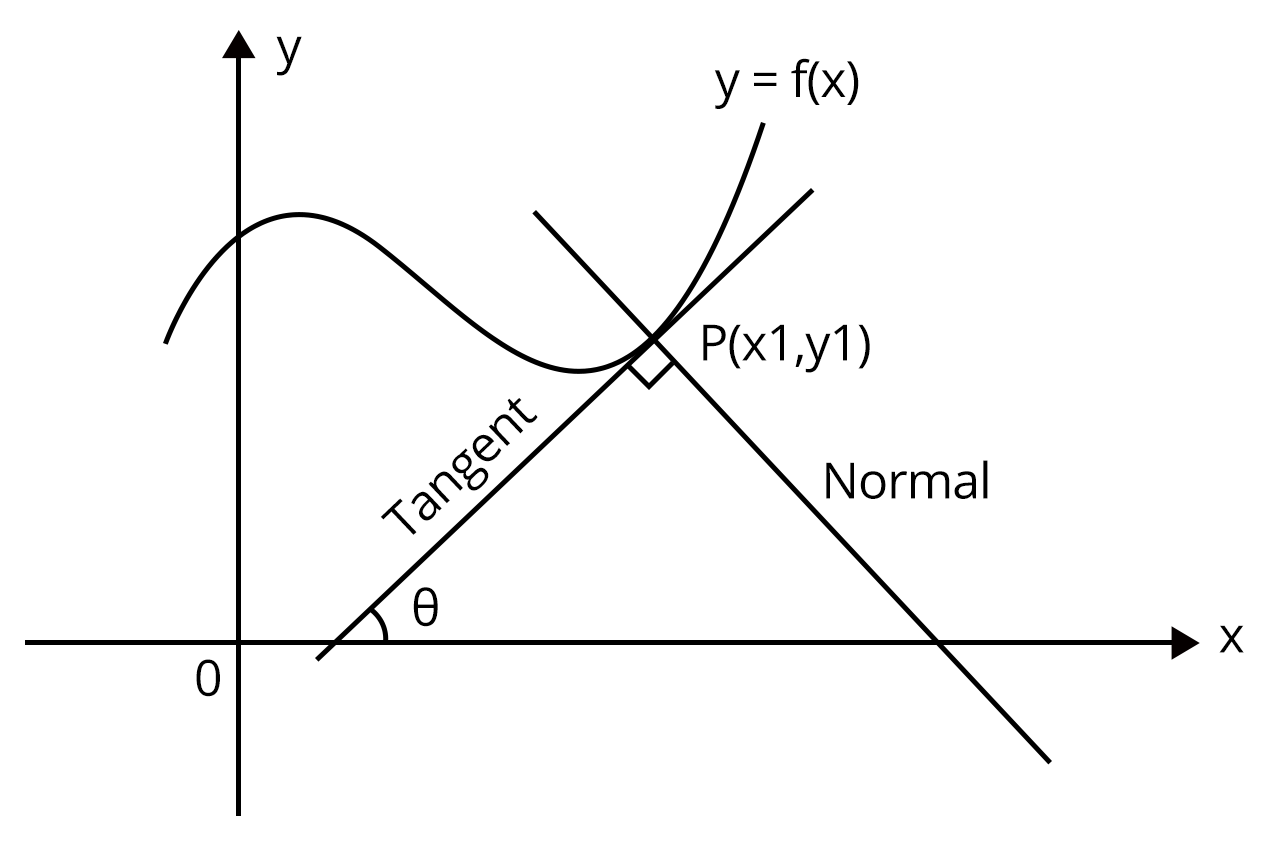If tangent at P makes an angle $\theta$ with positive direction of x -  axis, then slope of the tangent is $m = tan\theta$. Also, the slope of the tangent to the curve at any point on it is $\frac{dy}{dx}$.

$\therefore m = tan\theta =\frac{dy}{dx}$.

Also,the slope of normal is $-\frac{dx}{dy}$.

Therefore, equation of tangent at point $P\left ( x_{1}, \,\ y_{1} \right )$

$\Rightarrow \left ( y-y_{1} \right )= \frac{dy}{dx}_{\left ( x_{1}, \,\ y_{1} \right )}\left ( x_{1}, \,\ y_{1} \right )$.

And the equation of the normal at point $P\left ( x_{1}, \,\ y_{1} \right )$

$\Rightarrow \left ( y-y_{1} \right )= -\frac{dx}{dy}_{\left ( x_{1}, \,\ y_{1} \right )}\left ( x_{1}, \,\ y_{1} \right )$.

### Approximation Using Derivative

Consider the following figure to recall the definition of derivatives of function $y = f(x)$ at some point $P\left ( x, \,\ f(x) \right )\,\ w.r.to..x$.

From the definition of derivatives, we have

$\frac{dy}{dx}=\displaystyle \lim_{x \to 0}\frac{\Delta y}{\Delta x}= \displaystyle \lim_{x \to 0}\frac{f\left ( x+\Delta x \right )-f(x)}{\Delta x}$.

Here, when point Q moves closer to point P on the curve, then though $\Delta x$ does not tend to zero, $\Delta y$.

In that case,

$\Rightarrow \frac{dy}{dx}=\frac{\Delta y}{\Delta x}= \frac{f\left ( x+\Delta x \right )-f(x)}{\Delta x}$.

$\therefore f\left ( x+\Delta x \right )=f(x)+\Delta x \,\ \frac{dy}{dx}$.

### Monotonicity

One of the most important tools in analyzing the functions is their monotonic behavior. Functions like $f(x)= 2x+3, \,\ g(x)= log_{e}x \,\ and \,\ h(x) = x^{3}$ increase with the increase in the input value of the independent variable in their entire domain, i,e., graph of the function rises from left to right. Functions like $f(x)= cos^{-1}x$ and $g(x)= 0.5^{x}$ always decrease with increase in the value of x. Both of the above categories of functions are called monotonic functions.

Classification of functions based on monotonicity

• Increasing Function

• Strictly Increasing Function

• Decreasing Function

• Strictly Decreasing Function

### Maxima and Minima

Consider the graph of one differential functions as shown in the following figure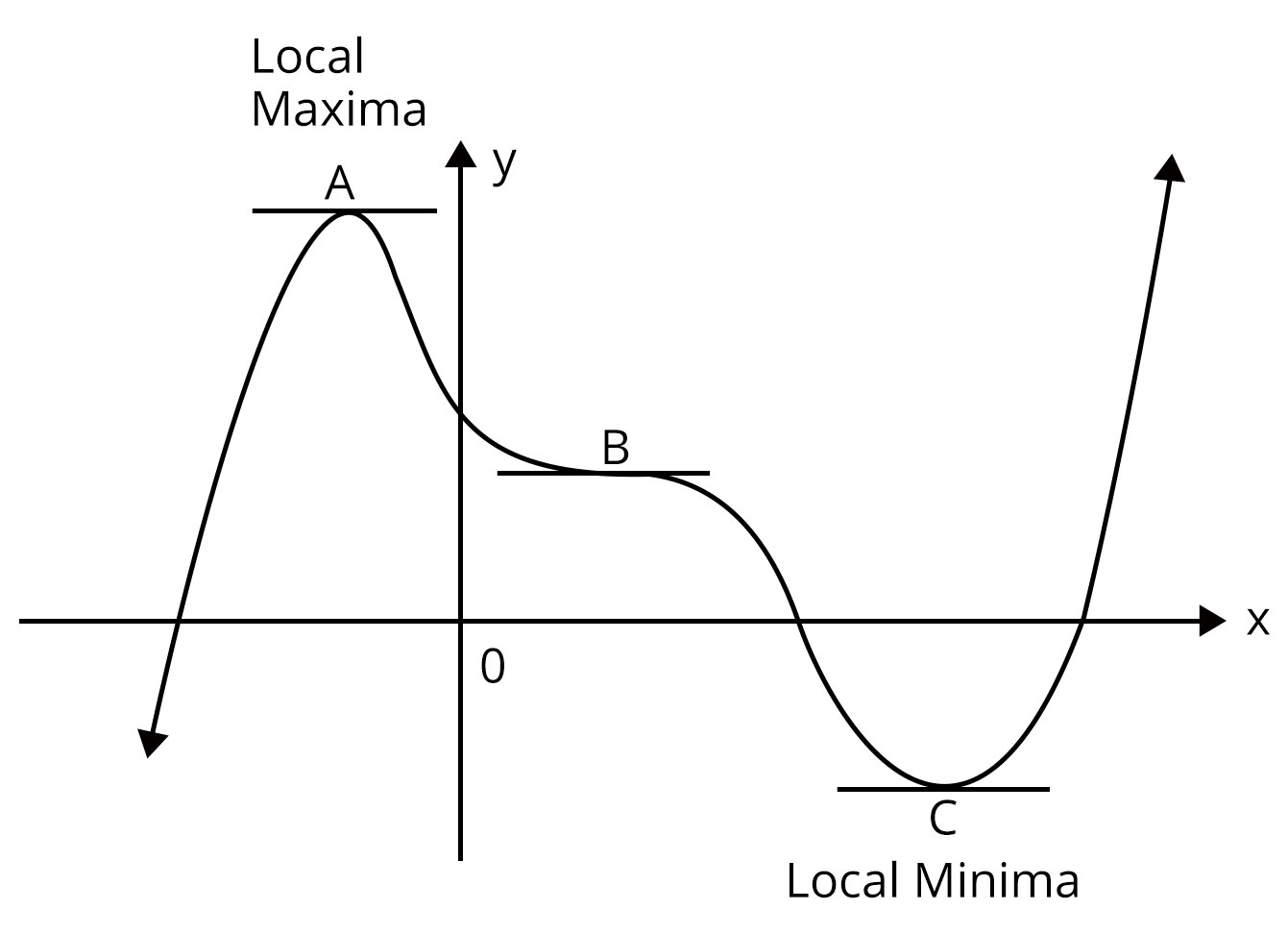We observe that points A, B and C are stationary points where tangent to the curve is parallel to the x axis i.e., the derivative is zero. These two stationary points are referred to as turning points.

Point A in figure is called a point of local maxima because in its immediate area it is the highest point, and so represents the greatest or maximum value of the function in that area.

Point B is called a local minimum because in its immediate area it is the lowest point, and so represents the least or minimum value of the function in that area.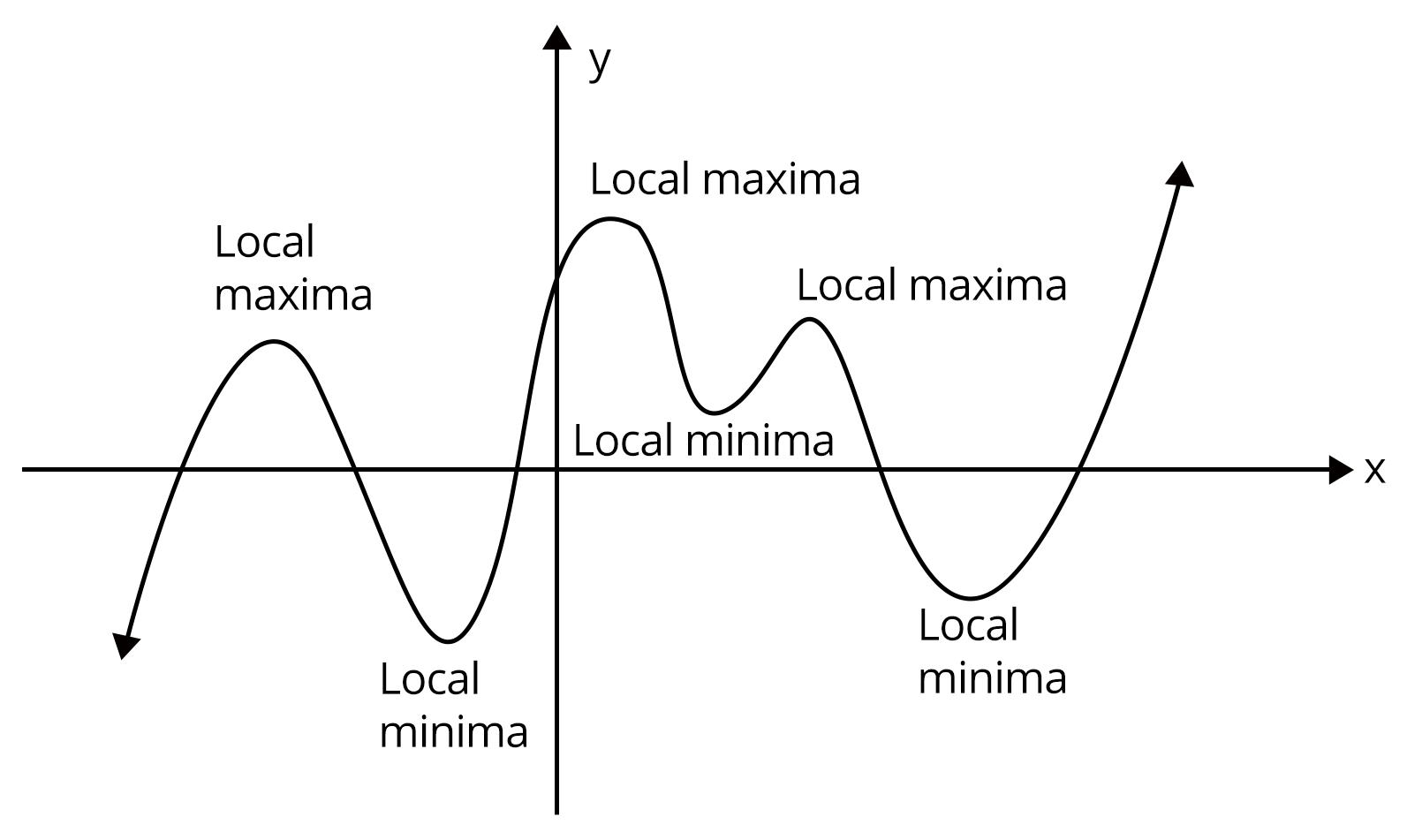### Solved Examples:

Example 1: If the equation of tangent to the curve $y^{2}=ax^{3}+b$ at point (2, 3) is y = 4x - 5, then find the values of a and b

Solution:

Here, we have  $y^{2}=ax^{3}+b$ ……………(1)

Differentiating w.r.t, x , we get

$\Rightarrow 2y\frac{dy}{dx}= 3ax^{2}$

$\Rightarrow \frac{dy}{dx}=\frac{3ax^{2}}{2y}$

$\left ( \frac{dy}{dx} \right )_{\left ( 2,3 \right )}=\frac{3\times a\times \left ( 2 \right )^{2}}{2\times 3}=2a$

Now, line y = 4x - 5 is tangent to the curve at (2, 3).

$\therefore 2a = 4$

$\Rightarrow a = 2$

Also, (2, 3) lies on the curve(1)

$\Rightarrow 9 = 8a+b$

$\therefore b=-7$

Example 2: If the graph of the function $f(x) = 3x^{4}+2x^{3}+ax^{2}-x+2$ is concave upward for all real x, then find the values of a.

Solution:

Here, we have $f'(x) = 12x^{3}+6x^{2}+2ax-1$

And $f''(x) = 36x^{2}+12x+2a$

Now graph of concave upward for all real x.

$\therefore f''(x)=36x^{2}+12x+2a>0$ for all real x.

Then, discriminant, $12^{2}-4(36)(2a)<0$

$\Rightarrow 1-2a<0$

$\Rightarrow a >\frac{1}{2}$

### Solved Problems of Previous Year Question

1. Let f, $f,g:\left [ -1,2 \right ]\to R$ be continuous functions which are twice differentiable on the interval (-1, 2). Let the values of f and g at the points -1, 0 and 2 be as given in the following table:

 x = -1 x=0 x=2 f(x) 3 6 0 g(x) 0 1 -1

In each of the intervals (-1, 0) and (0, 2), the function (f-3g)’’ never vanishes. Then the correct statements is are

1. $f'(x)-3g'(x)=0$ has exactly three solutions in $\left ( -1,0 \right )\cup \left ( 0,2 \right )$.

2. $f'(x)-3g'(x)=0$ has exactly one solution in (-1, 0)

3. $f'(x)-3g'(x)=0$ has exactly one solution in (0, 2)

4. $f'(x)-3g'(x)=0$ has exactly two solutions in (-1, 0) and exactly two solutions in (0, 2)

Ans: (b), (c)

Let $h(x)=f(x)-3g(x)$

$h(-1)= f(-1)-3g(-1)=3-0=3$

$h(0)= f(0)-3g(0)=6-3=3$

$h(2)= f(2)-3g(2)=0-(-3)=3$

Thus, $h'(x)= 0$ has at least one root in (-1 , 1) and at least one root in (0, 2).

But Since $h''(x)= 0$ has no root in (-1, 0) and (0, 2) therefore $h'(x)= 0$ has exactly 1 root in (-1, 0) and exactly 1 root in (0, 2).

2. The function $f(x)=2\left|x \right|+\left|x +2 \right|+\left|\left|x+2 \right|-2\left|x \right| \right|$ has a local minimum or a local maximum at x =

1. $-2$

2. $\frac{-2}{3}$

3. $2$

4. $\frac{2}{3}$

$\Rightarrow \frac{f(x)=2\left|x \right|+\left|x +2 \right|+\left|\left|x+2 \right|-2\left|x \right| \right|}{2}=Min \, \left ( \left| 2x\right|, \left|x+2 \right| \right )$.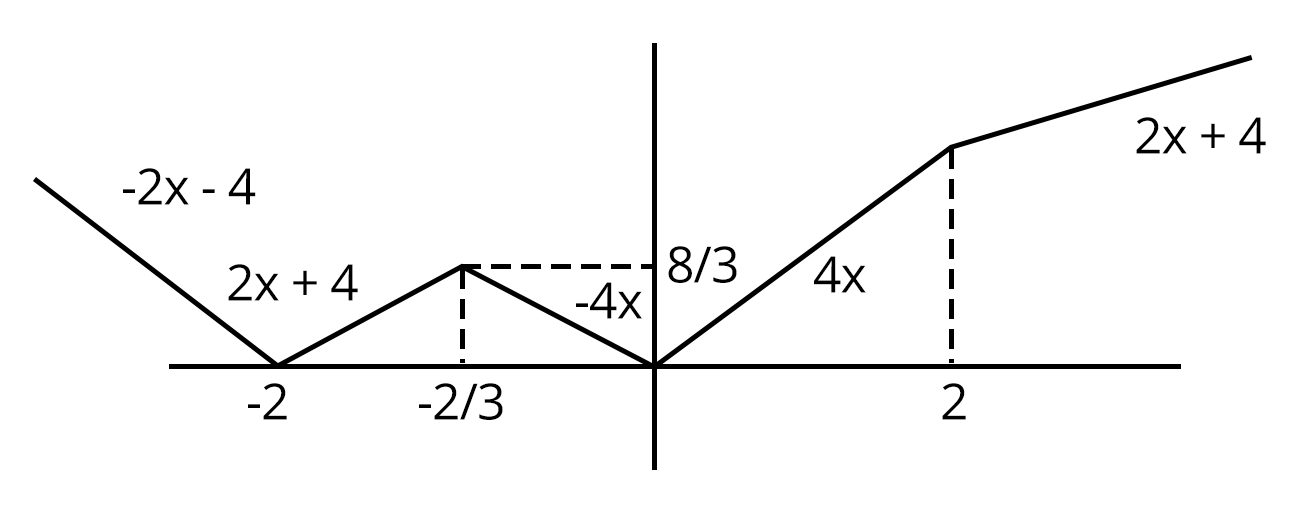From the figure shown, points of local minima/ maxima are x = - 2, -⅔. 0

3. Let $a\in R$ and let $f:R\to R$ be given by $f(x)=x^{5}-5x+a$, then

1. f(x) has three real roots if a > 4

2. f(x) has only one real root if a > 4

3. f(x) has three real roots if a < - 4

4. f(x) has three roots if - 4 < a < 4

Ans: b, d

Let $y= f(x)=x^{5}-5x$

$\Rightarrow f'(x)= 5x^{4}-5$

$= 5(x-1)(x+1)(x^{2}+1)$

$f'(x)=0, \, \therefore x=-1,1$

$f''(x)= 20x^{3}$

$f''(1)= 20$ and $f''(-1)= -20$

Graph of y = f(x) is as shown in the following figure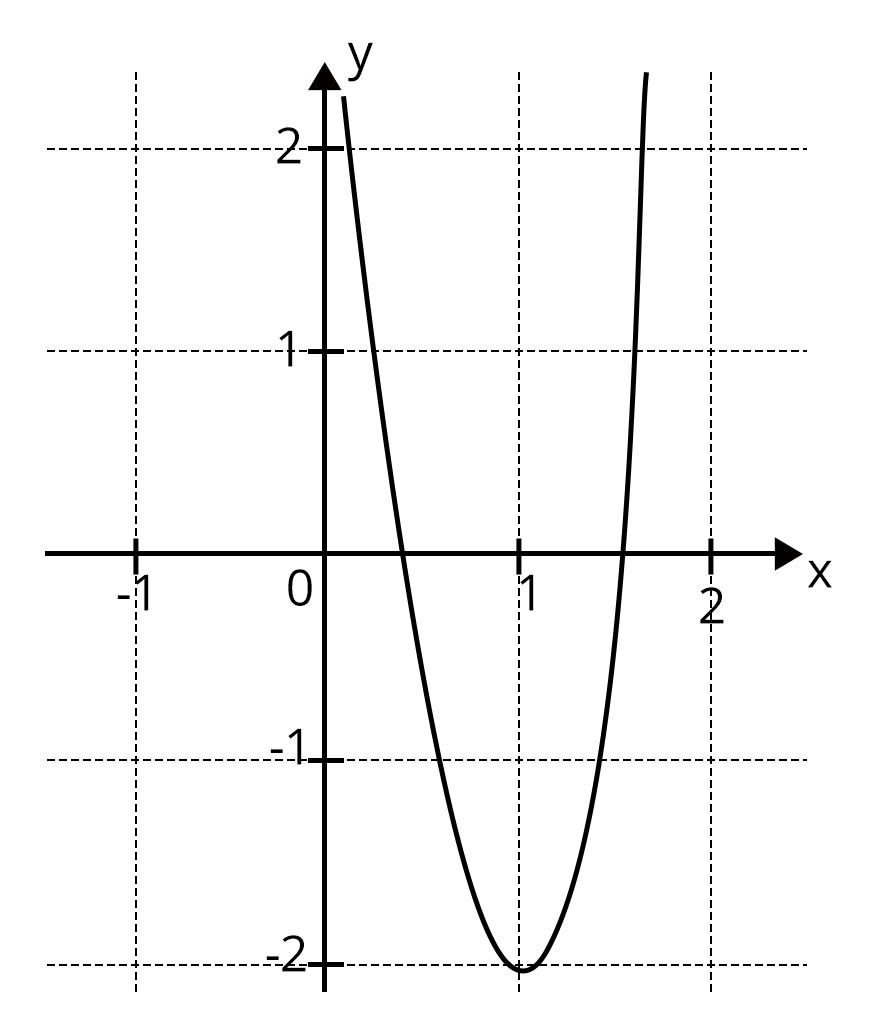From the graph $x^{5}-5x=-a$ has one real root if $-a<-4$ or $-a>4$

$\Rightarrow a>4 \,\ or \,\ a<-4$

$x^{5}-5x = -a$ has three real roots if -4 < -a < 4 i.e., -4 < a < 4

### Practice Question:

1. If f : R is a differentiable function such that $f'(x)> 2f(x)$ for all $x\in R$, and f(0) = 1, then

1. $f(x)>e^{2x}$ in $\left ( 0, \infty \right )$

2. f(x) is decreasing in $\left ( 0, \infty \right )$

3. f(x) is increasing in $\left ( 0, \infty \right )$

4. $f'(x)<e^{2x}$ in $\left ( 0, \infty \right )$

2. The function $f : \left [ 0, 3 \right ]\to \left [ 1, 29 \right ]$ defined by $f(x)= 2x^{3}-15x^{2}+36x+1$, is

1. One - one and onto

2. Onto but not one one

3. One - one but not onto

4. Neither one - one nor onto

### Conclusion:

In this chapter, we have elaborated on concepts and solutions to questions on the topic of Differential Calculus.  Everything you're looking for is available in a single location. Students can carefully read through the Concepts, Definitions, and questions in the PDFs, which are also free to download and understand the concepts used to solve these questions. This will be extremely beneficial to the students in their exams.

See MoreView All Dates
JEE Advanced 2022 exam date and revised schedule have been announced by the NTA. JEE Advanced 2022 will now be conducted on 28-August-2022, and the exam registration closes on 11-August-2022. You can check the complete schedule on our site. Furthermore, you can check JEE Advanced 2022 dates for application, admit card, exam, answer key, result, counselling, etc along with other relevant information.
See More
AugustRelease of Application form
07-Aug-2022Last date to fill the form
11-Aug-202223-Aug-202228-Aug-202203-Sep-202203-Sep-202211-Sep-2022Declaration of Result
11-Sep-2022
View All DatesApplication Form
Eligibility Criteria
Reservation Policy
IIT Bombay has announced the JEE Advanced 2022 application form release date on the official website https://jeeadv.ac.in/. JEE Advanced 2022 Application Form is available on the official website for online registration. Besides JEE Advanced 2022 application form release date, learn about the application process, steps to fill the form, how to submit, exam date sheet etc online. Check our website for more details.
Book your Free Demo session
Get a flavour of LIVE classes here at VedantuVedantu Improvement Promise
We promise improvement in marks or get your fees back. T&C Apply*View JEE Advanced Syllabus in Detail
It is crucial for the the engineering aspirants to know and download the JEE Advanced 2022 syllabus PDF for Maths, Physics and Chemistry. Check JEE Advanced 2022 syllabus here along with the best books and strategies to prepare for the entrance exam. Download the JEE Advanced 2022 syllabus consolidated as per the latest NTA guidelines from Vedantu for free.
See More
Paper 1
Paper 2MathsPhysics
View JEE Advanced Syllabus in Detail## JEE Advanced 2022 Study Material

View all study material for JEE Advanced
JEE Advanced 2022 Study Materials: Strengthen your fundamentals with exhaustive JEE Advanced Study Materials. It covers the entire JEE Advanced syllabus, DPP, PYP with ample objective and subjective solved problems. Free download of JEE Advanced study material for Physics, Chemistry and Maths are available on our website so that students can gear up their preparation for JEE Advanced exam 2022 with Vedantu right on time.
See More
All
Chemistry
Maths
Physics
See Allsee all
See More
PYQP
Sample Paper
2021
All Years
Paper 1
24th July 2021 - physicsEnglish  •   Shift 1views
24th July 2021 - chemistryEnglish  •   Shift 1views
30th July 2021 - mathematicsEnglish  •   Shift 1views
Paper 2
30th July 2021 - physicsEnglish  •   Shift 2views
04th August 2021 - chemistryEnglish  •   Shift 2views
04th August 2021 - mathematicsEnglish  •   Shift 2views
Paper 1
mathematicsEnglish  •   Shift NAviews
mathematicsEnglish  •   Shift NAviews
mathematicsEnglish  •   Shift NAviews
mathematicsEnglish  •   Shift NAviews
mathematicsEnglish  •   Shift NAviews
Paper 2
mathematicsEnglish  •   Shift NAviews
mathematicsEnglish  •   Shift NAviews
mathematicsEnglish  •   Shift NAviews
mathematicsEnglish  •   Shift NAviews
mathematicsEnglish  •   Shift NAviewsView all JEE Advanced Important Books
In order to prepare for JEE Advanced 2022, candidates should know the list of important books i.e. RD Sharma Solutions, NCERT Solutions, HC Verma books and RS Aggarwal Solutions. They will find the high quality readymade solutions of these books on Vedantu. These books will help them in order to prepare well for the JEE Advanced 2022 exam so that they can grab the top rank in the all India entrance exam.
See MoreMaths
NCERT Book for Class 12 MathsPhysics
NCERT Book for Class 12 Physics
See AllIIT Bombay is responsible for the release of the JEE Advanced 2022 cut off score. The qualifying percentile score might remain the same for different categories. According to the latest trends, the expected cut off mark for JEE Advanced 2022 is 50% for general category candidates, 45% for physically challenged candidates, and 40% for candidates from reserved categories. For the general category, JEE Advanced qualifying marks for 2021 ranged from 17.50%, while for OBC/SC/ST categories, they ranged from 15.75% for OBC, 8.75% for SC and 8.75% for ST category.
See More## Toppers## Master TeachersFrom IITs & other top-tier colleges with 5+ years of experienceYou can count on our specially-trained teachers to bring out the best in every student.They have taught over 4.5 crore hours to 10 lakh students in 1000+ cities in 57 countries11+ years exp

### Shreyas

Physics master teacher

#### NIT Nagpur4+ years exp

### Nidhi Sharma

Chemistry master teacher

#### Jamia Hamdard2+ years exp

### Luv Mehan

Chemistry Master Teacher

#### IISc BangaloreIIT Bombay is responsible for the release of the JEE Advanced 2022 cut off score. The qualifying percentile score might remain the same for different categories. According to the latest trends, the expected cut off mark for JEE Advanced 2022 is 50% for general category candidates, 45% for physically challenged candidates, and 40% for candidates from reserved categories. For the general category, JEE Advanced qualifying marks for 2021 ranged from 17.50%, while for OBC/SC/ST categories, they ranged from 15.75% for OBC, 8.75% for SC and 8.75% for ST category.
See MoreThe JEE Advanced 2022 result will be published by IIT Bombay on https://jeeadv.ac.in/ in the form of a scorecard. The scorecard will include the roll number, application number, candidate's personal details, and the percentile, marks, and rank of the candidate. Only those candidates who achieve the JEE Advanced cut-off will be considered qualified for the exam.
See More
Rank List
Counselling
Cutoff
JEE Advanced 2022 State Wise Rank List - The respective state authorities started releasing the JEE Advanced 2022 state merit lists for admission to Engineering course. Check JEE Advanced 2022 state-wise rank list for the year 2022 here.Want to know which Engineering colleges in India accept the JEE Advanced 2022 scores for admission to Engineering? Find the list of Engineering colleges accepting JEE Advanced scores in India, compiled by Vedantu. There are 1622 Colleges that are accepting JEE Advanced. Also find more details on Fees, Ranking, Admission, and Placement.
See MoreFAQ

1. What are some applications of Derivatives in real-life examples?

Almost all the applications have some real-life usage when it comes to partial derivatives and absolute derivatives. When a value y varies with x such that it satisfies y=f(x), then f’(x) = dy/dx is called the rate of change of y with respect to x. Also, f’(x0) = dy/dx x=x0 is the rate of change of y with respect to x=x0. The rate of change of values is a significant application of differentiation, which is used broadly in physics and other engineering subjects.

2. What is the definition of tangent in Mathematics?

The tangent is a straight line that just touches the curve at a given point. The normal is a straight line that is perpendicular to the tangent. The tangent to any curve at a given point is observed to have the same gradient as the curve at that point. A derivative is required in order to find the gradient of the curve, which can be found by differentiating and obtaining an expression for dy/dx. The gradient of the tangent can be achieved by substituting an x-value. To find the full equation of the tangent, it remains to find the y-intercept of the tangent, which can be found using the coordinates of the point that the tangent and the curve have in the common.Last date for submitting JEE Advanced application form
12 Sep 202274 days remaining28 Sep 202290 days remaining## Notice board

News
BlogJEE Main 2022: A Few Hyderabad Centres Witnessed Server Issues
24 Jun 2022JEE Main 2022: Day 2 Shift 1 Exam Analysis
23 Jun 2022